# AP Physics B : Waves

## Example Questions

### Example Question #1 : Ap Physics 2

In the electromagnetic spectrum, __________ have the highest frequency and __________ have the greatest energy per photon.

gamma rays . . . gamma rays

X-rays . . . radio waves

ultraviolet light . . . X-rays

infrared light . . . ultraviolet light

microwaves . . . microwaves

gamma rays . . . gamma rays

Explanation:

The relationship between frequency and energy is determined by Planck's constant in a direct relationship. This means that as frequency increases, energy increases as well.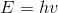The electromagnetic waves with the highest frequency will have the greatest energy per photon, and vice versa.

In the electromagnetic spectrum, radio waves have the lowest frequency (largest wavelength) and gamma rays have the highest frequency (shortest wavelength). Gamma rays will thus have the highest frequency and greatest energy.

### Example Question #1 : Ap Physics 2

Two in-phase waves travelling in the same direction with the same period have amplitudes of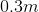and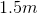, respectively. A third, out-of-phase wave with the same period as the other two has an amplitude of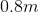. What will be the amplitude of the final resultant wave when these three are aligned?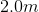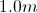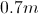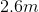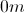Explanation:

The amplitudes of in-phase waves add in what is called constructive interference. We can find the resultant amplitude of the first two waves by adding their individual amplitudes.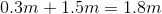The third wave is out of phase, meaning that it will contribute destructive interference when the three waves align. We subtract the amplitude of the third wave from the total of the first two.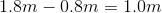This is the final amplitude of the resultant wave.